# November 04, 2017

## Impulse Noise Filtering - White Noise vs Impulse Noise

### Impulse Noise Filtering

There are two main types of noise one encounters in the embedded software world. They are white noise and impulse noise. Let’s start with a perfect signal.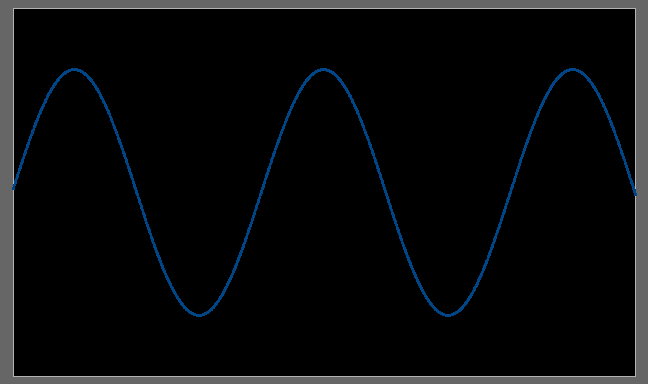Here is a plain sine wave with no distortion. For all examples, this is the actual signal. We will start with white noise. This is a mainly uniform random addition to the signal of varying amplitude.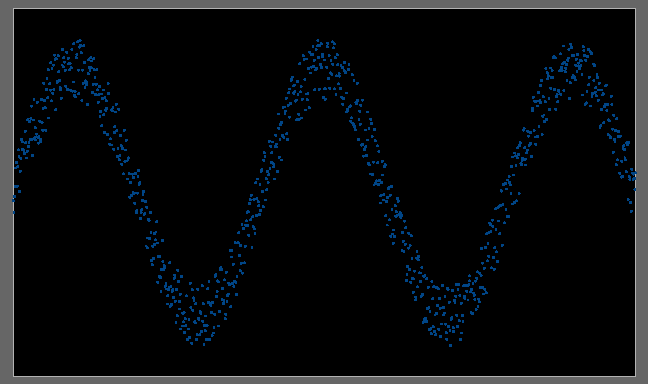Here we see the same signal but with the addition of white noise. Some amount of white noise is common in analog signals. Often a hardware low-pass filter is added to help remove it, but white noise can be the result of the analog to digital converter. Filtering such noise is easy with a software low-pass filter and I have written in the past about using a rolling average to do such filtering.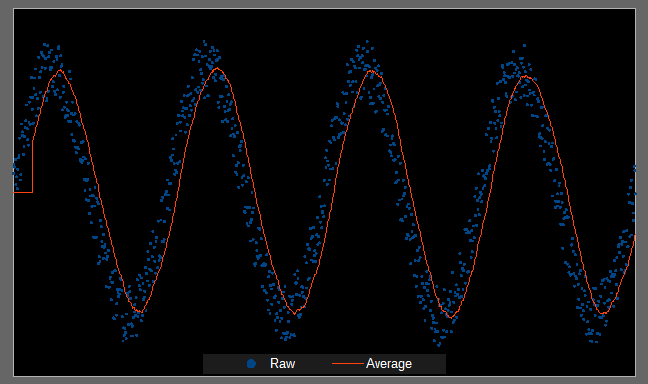This is a simple windowed average that has reconstructed the original sine wave. Note that because of the window size there is a lag in the beginning. Also the entire signal has been shifted (phase shift) to the right and the amplitude has been attenuated. These are artifacts of using this kind of low-pass filter. Typically these artifacts do not present too much of a problem but it really depends on the signal being filtered.

There are many sources of white noise and good hardware practices often reduce much of this noise before it ever gets to an analog to digital converter. Nonetheless this is the most common noise I encounter in embedded projects.

Another kind of noise electrical impulse noise. These are random rapid changes in the signal often called spikes. Unlike white noise the magnitude is more dramatic.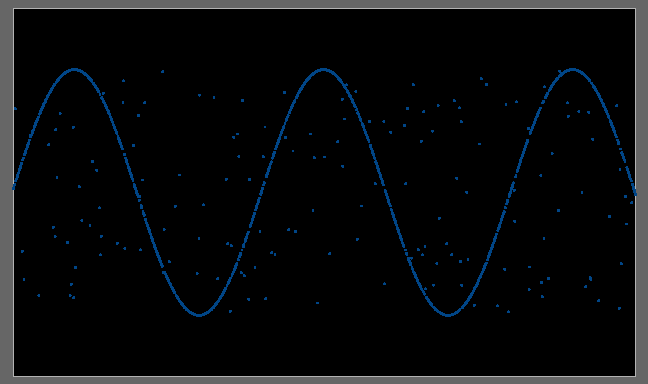This is an example of impulse noise. Filtering noise impulse noise is more difficult because the simple average low-pass filter isn’t as effective at removing this kind of noise.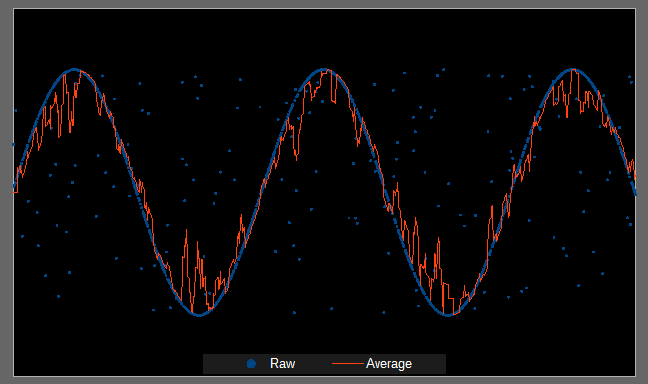Shown is a windowed-average low-pass filter being applied to the signal with impulse noise. Averages are thrown off by large deviations and thus the filtered signal is fairly messy.

One solution is to instead use a windowed median filter. It is a low-pass filter but using the median rather than the average. The median is simply the middle value of a set of sorted numbers and it is trivial to calculate. For example in the following set of number: 34, 96, 63, 74, 81. First sort the list: 34, 63, 74, 81, 96. Then pick the middle value which is 74.

Why this is useful in an impulse noise is because a large spike in values will always end up at the beginning or end of the sorted list, and because the median always chooses from the middle these outlier are always ignored. If we were to use a standard first-order low-pass filter (i.e. the average) this would not be the case. For example, say we have the following numbers: 34, 81, 63, 1000, 82. Clearly the large spike is the value 1000, which when sorted ends up as the highest value. The median of this set is 81 where as the average is 252.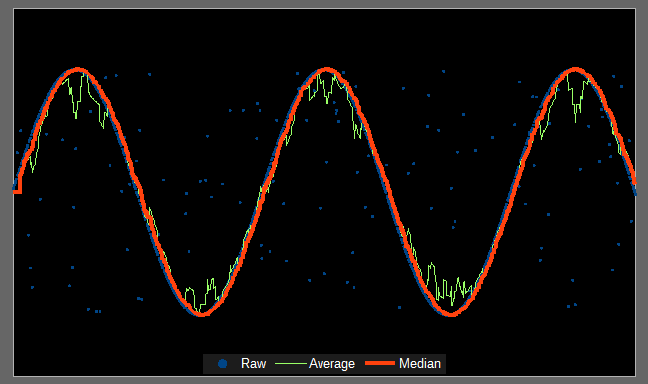This is a median filter in orange with the average filter in green. The median filter does a far better job of reconstructing the original signal. Median filters also introduce phase shift and signal attenuation. In addition they produce plateaus around local extrema.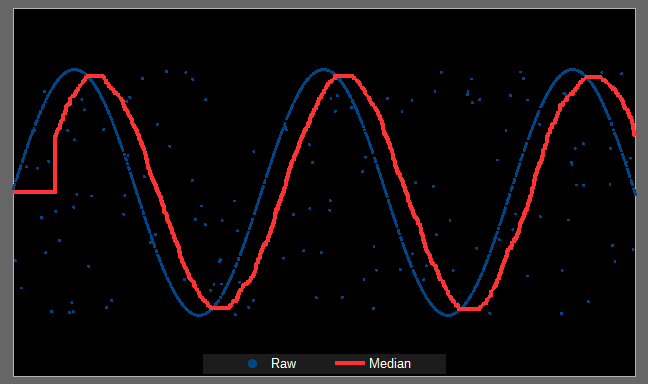This signal has a large filter window and has strong phase shift to the right, some signal attenuation, and the peaks are all plateaued. Again, these artifacts need to be understood before using a median filter. The smaller the filter window size, the less the effect. However, if the filter window is too small noise spikes can get through.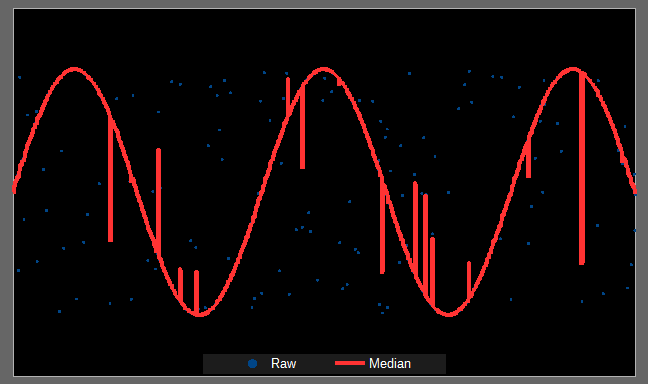Here the window size was too small and while many of the spikes have been removed, some have not.

In subsequent articles I will cover how to implement a median filter and give a demonstration of how it works.

# November 05, 2017

## Impulse Noise Filtering - Median Filter Demo

### Math Demonstration, Impulse Noise Filtering

In this demonstration there are three sliders. From left to right they are: signal noise, spike density, and filter window length. The signal noise is just basic white noise added to the signal. The spike density is the percentage of impulse noise added to the signal. And the filter window is how many samples are used by the median filter.

Shown in red is the raw signal, complete with spikes. In blue is the median filtered signal. The goal is to have the blue signal from a smooth trace with no spikes, and the default settings should produce this. Increasing the spike density will require a larger filter window.

Just as with an average filter, the median filter will induce phase shift as well as attenuate the signal. The larger the filter window the larger the phase shift and the more attenuation. Unique to the median filter is the creation of plateaus around signal peaks. This is because of the phase shift and the changing direction of the signal lags during peak values.

# November 06, 2017

## Impulse Noise Filtering - Implementation of Median Filter

### Programming, Impulse Noise Filtering

When implementing the median filter there are two options: off-line or real-time. If not done off-line then the filter loops though each data point and calculates the median over a window of the data. Here is an example with a buffer size of 5 and the median window size of 3:

 55 42 1000 86 61 55 42 1000 86 61 55 42 1000 86 61

Taking the median for the number highlighted in blue for each row, the filtered data would then be:

 55 86 86

Note how the spike of 1000 is filtered out. Note also the resulting buffer is shorter. The filtered data size is always the initial data size less the window size.

If running the filter in real-time, there needs to be a ring-buffer that holds the window size samples. The filtered result is the median of this buffer. New samples replace old samples and the filter continues. Starting this filter requires either loading some starting values or waiting until enough samples have been taken to fill the buffer.

If the following is the initial ring buffer at start:

 21 30 71 62 72

Then the first output is 62. Assume the current into into the buffer is the second sample. When a new sample arrives, it will replace the second sample. For instance if the new sample is 41, the ring buffer becomes:

 21 41 71 62 72

The median of this is again 62 which is the output of the filter. In this way the filter can be used in real-time.

Getting the filter started is up to the implementer. Most of the time simply using an initial buffer full of zeros works just fine. One can also delay producing filtered output until enough samples have been acquired. The entire buffer can be loaded entirely with the first sample taken. Or the window used for calculations can gradually grow until it reaches the desired size. Which option used depends on the application.

Basic implementation of the median calculation itself is simple. Sort the data and choose the middle data point. However sorting data is a time consuming task and fast sorting algorithms don’t have fixed run times. In addition if one is using a ring-buffer to hold samples the sorting cannot be done in place.

The easiest filter to implement is a selection sort. It is simple and always takes the same number of steps for any window size. Selection sort is also the slowest sort with a fixed complexity of O( n2 ). One bit of acceleration can be gained by only sorting half the data. Since the median is looking for the middle data point in a sorted set, one needs only to find the middle data point—everything else is ignored.

My co-worker Flux suggested I look into using an insertion sort for small filter window sizes. Worst case an insertion sort has the same speed, O( n2 ) as a selection sort. However the speed improves the more sorted the data initially is. If already sorted the speed of the algorithm is O( n ). Since we are running as a windowed filter we are only ever changing one piece of data at a time. Thus the data remains mostly sorted. As a result the insertion sort runs closer to O( n ) complexity and worst case O( 2n )—once through the array, and once again needing to move the last element to the first element. That allows this filter to still be useful in real-time applications, especially for small window sizes.

Here is a simple C implementation of a median filter useful for real-time applications.

//-----------------------------------------------------------------------------
// Uses:
//   Return the median of the data buffer.
// Input:
//   data - Data from which to select median.
//   indexes - Buffer to hold sort indexes (must contain 'windowSize' elements).
//   windowSize - Number of data points.
// Output:
//   Median of data points.
// Notes:
//   Designed for small data sizes.
//   The 'indexes' must be pre-initialized at least once.  After
//   initialization it can be used for all subsequent calls of the same
//   window size.
//       for ( int index = 0; index < windowSize; index += 1 )
//         indexes[ index ] = index;
// Author:
//   Andrew Que <https://www.DrQue.net/>
//-----------------------------------------------------------------------------
static inline int median
(
int const * data,
int * indexes,
int windowSize
)
{
// Insert sort the data using swap buffer.
for ( int indexA = 1; indexA < windowSize; indexA += 1 )
{
int value = data[ indexes[ indexA ] ];
int swapIndex = indexes[ indexA ];

int indexB = indexA - 1;
while ( ( indexB >= 0 )
&& ( data[ indexes[ indexB ] ] > value ) )
{
indexes[ indexB + 1 ] = indexes[ indexB ];
indexB -= 1;
}

indexes[ indexB + 1 ] = swapIndex;
}

// Return median.
return data[ indexes[ windowSize >> 1 ] ];
}

The code is designed for a ring-buffer and requires a second buffer to hold indexes. One should replace all int with the necessary word size for the application but otherwise the code should be drop-in. The algorithm is fairly efficient up to a few tens of window size. After that some more efficient sorting techniques should be used.

# November 16, 2017

## Impulse Noise Filtering - Slew Limit Filter

### Math, Programming, Impulse Noise Filtering

In the past few articles in this series I have demonstrated using a median filter to mitigate impulse noise. The problem with a median filter is that even using a small window size and taking advantage of the speed of a mostly sorted list entering an insertion sort, the filter is still significantly slower than a windowed average. There is another option. It does not filter as nicely as a median filter but does help to dampen impulse noise.

The slew rate is a measure of how quickly voltage changes over time. A windowed average acts as limiter on slew rate but usually not enough to attenuate impulse noise. However one can directly limit the slew rate of a signal. The equation is quite simple: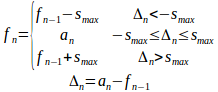For each sample of the input (an) the filter output (fn) has its change (Δn) limited to the last to some maximum (smax). If the magnitude of change is less than the maximum, the filter output is the same as the input. If the magnitude exceeds the change the filtered output is modified by the maximum change but no further.

Following the examples of previous article on this subject, here is an example of the filter output: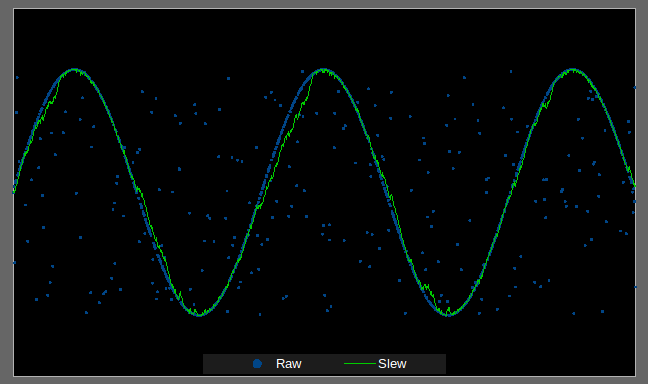There is some distortion on the signal caused by the impulse noise but the filtered output is fairly effective at eliminating the large spikes. When used in combination with a first-order low-pass filter the input signal is fairly clear.

The reason this kind of filter is attractive is because of how simple it is to implement.

//-----------------------------------------------------------------------------
// Uses:
//   In-place filter of input buffer.
// Input:
//   data - Data to filtered..
//   dataSize - Number of data points.
//   limit - Maximum change allowed.
// Output:
//   Filtered output is returned in 'data'.
// Author:
//   Andrew Que <https://www.DrQue.net/>
//-----------------------------------------------------------------------------
static inline void slewLimitFilter
(
int * data,
size_t dataSize,
int limit
)
{
for ( size_t index = 1; index < dataSize; index += 1 )
{
int delta = data[ index ] - data[ index - 1 ];
if ( delta > limit )
data[ index ] = data[ index - 1 ] + limit;
else
if ( delta < -limit )
data[ index ] = data[ index - 1 ] - limit;
}
}

Just as with any filter, artifacts are introduced by the slew limit filter.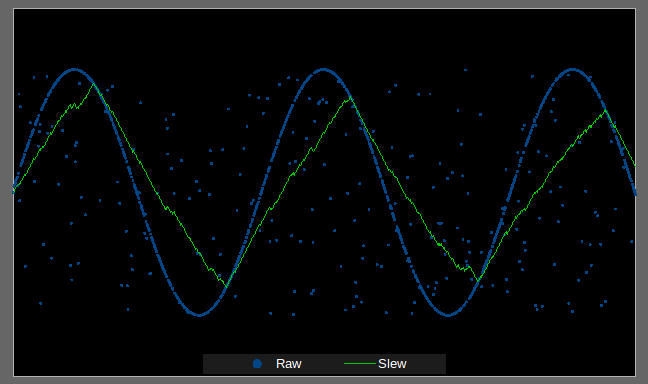This sine wave has become a triangle wave because the slew limit is too low. This shape is the result of the filter attenuating the actual data signal. This is an other demonstration.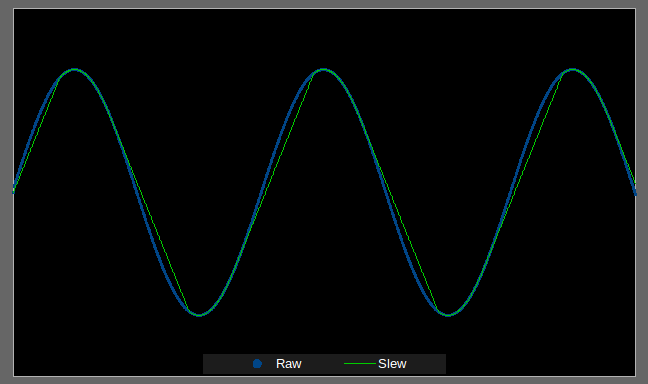Here is a clean signal, but because the slew limit is too low the filter cannot keep up with the rate of change of the true signal.

The other problem with the slew limit filter is that it is not very strong.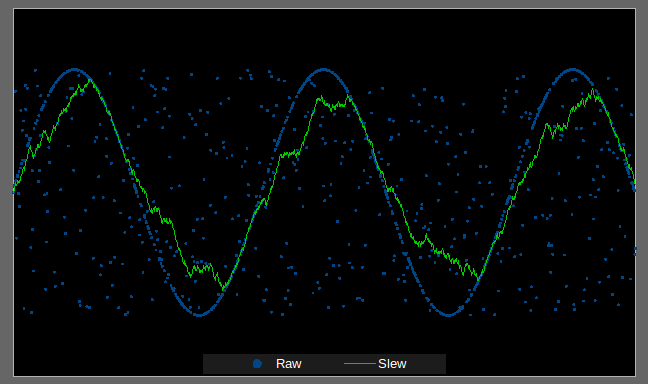Here the impulse noise has been doubled and the filter has produced a very messy result. So the filter is clearly not a replacement for a median filter. If you can afford the processing power, use the median filter. If you cannot, the slew limit filter may be a possibility. It depends on what the impulse noise looks like, and how much signal distortion can be tolerated.